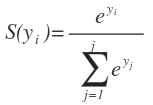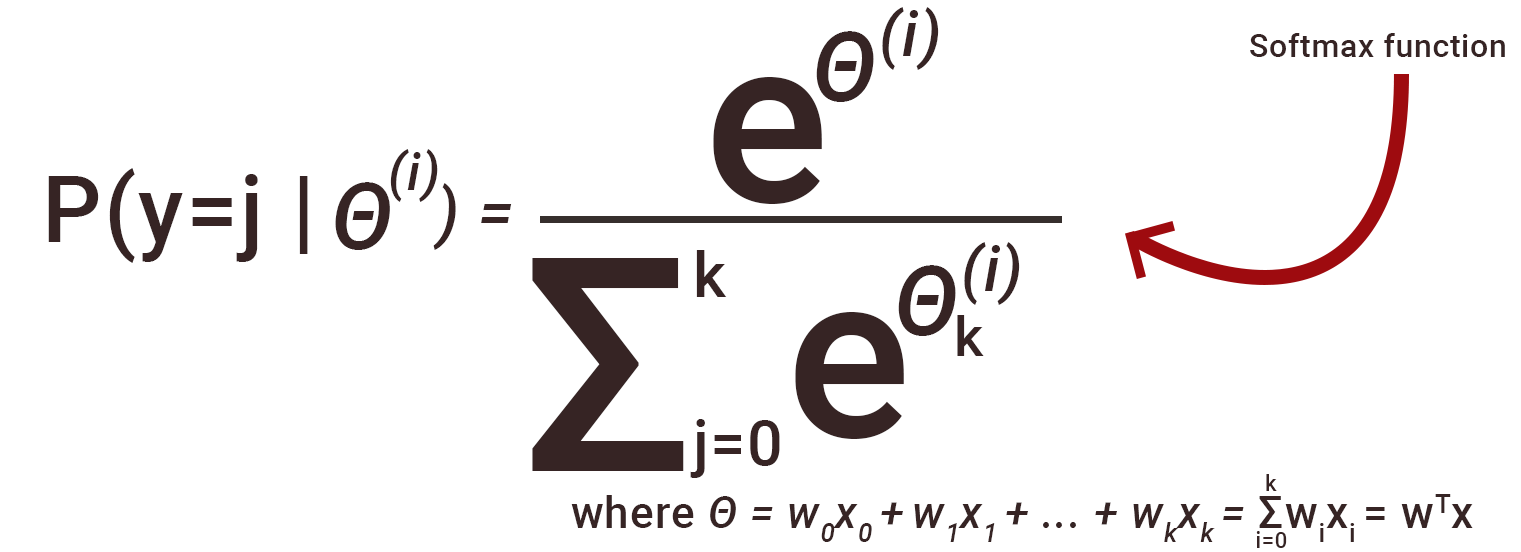2 views

Question:

There are many activation functions in machine learning. I’m unable to understand the softmax function. Softmax function calculates the sum of exponentials, but how can we use softmax function as an activation function? How to implement softmax function in python?Where S(y_i) is the softmax function of y_i and e is the exponential and j is the no. of columns in the input vector Y.

I've tried the following:

import numpy as np

def softmax(x):

"""Compute softmax values for each sets of scores in x."""

e_x = np.exp(x - np.max(x))

return e_x / e_x.sum()

scores = [3.0, 1.0, 0.2]

print(softmax(scores))

which returns:

[ 0.8360188 0.11314284 0.05083836]

But the suggested solution was:

def softmax(x):

"""Compute softmax values for each sets of scores in x."""

return np.exp(x) / np.sum(np.exp(x), axis=0)

which produces the same output as the first implementation, even though the first implementation explicitly takes the difference of each column and the max and then divides by the sum.

Can someone show mathematically why? Is one correct and the other one wrong?

Is the implementation similar in terms of code and time complexity? Which is more efficient?

closed

by (33.1k points)
edited by

The softmax function is an activation function that turns numbers into probabilities which sum to one. The softmax function outputs a vector that represents the probability distributions of a list of outcomes. It is also a core element used in deep learning classification tasks.

• Softmax function is used when we have multiple classes.

• It is useful for finding out the class which has the max. Probability.

• The Softmax function is ideally used in the output layer, where we are actually trying to attain the probabilities to define the class of each input.

• It ranges from 0 to 1.

Softmax function turns logits [2.0, 1.0, 0.1] into probabilities [0.7, 0.2, 0.1], and the probabilities sum to 1. Logits are the raw scores output by the last layer of a neural network. Before activation takes place. To understand the softmax function, we must look at the output of the (n-1)th layer.

The softmax function is, in fact, an arg max function. That means that it does not return the largest value from the input, but the position of the largest values.

For example:

Before softmax

X = [13, 31, 5]

After softmax

array([1.52299795e-08, 9.99999985e-01, 5.10908895e-12]

The sum of outputs after the softmax function, can’t be 100%, because neural networks are universal function approximators. We can build a neural network that approximates the value of any mathematical function, but that is just an approximation, not an exact result. We use softmax to embrace that uncertainty and turn it into a probability interpretable by people.Code:

import numpy as np

def your_softmax(x):

"""Compute softmax values for each sets of scores in x."""

e_x = np.exp(x - np.max(x))

return e_x / e_x.sum()

# correct solution:

def softmax(x):

"""Compute softmax values for each sets of scores in x."""

e_x = np.exp(x - np.max(x))

return e_x / e_x.sum(axis=0)

# only difference

If you want to learn Python for Data Science then you can watch this complete video tutorial: